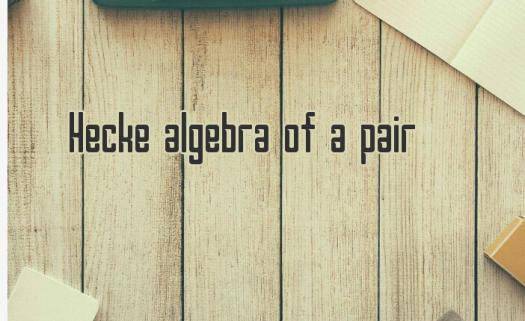# What Do You Know About The Hecke Algebra Of A Pair?

10 Questions | Total Attempts: 104SettingsHecke algebra of a pair, or Hecke pair as it is shortly called, consists of an approximate pair of identity, whose approximate modules of unital value are the same as the K-finite representation of the pairs. The algebra usually appears with k being a compact subgroup of a Lie group that has a Lie algebra g. To properly understand Hecke algebra, there is a need for certain preliminary knowledge. This short quiz will give some idea about Hecke algebra in general.

• 1.
Hecke algebra was studied by who?
• A.

Erich Hecke

• B.

Hecke Iwahori

• C.

Schur Hecke

• D.

Erich Schur

• 2.
Hecke algebra has which of the following subgroups?
• A.

• B.

G

• C.

F

• D.

X

• 3.
How can the algebra also be interpreted? An algebra of...
• A.

Minor cosets

• B.

Major cosets

• C.

Subsets

• D.

Double cosets

• 4.
Who studied Hecke ring?
• A.

Ian Grey

• B.

Ian McDonald

• C.

George Lusztig

• D.

None of the above

• 5.
When we start with a Gelfrand pair in Hecke algebra, what is the resulting algebra called?
• A.

• B.

Gelfrand equation

• C.

Commutative

• D.

Hecke equation

• 6.
Which of the following is a finite-dimensional smooth manifold G with a group of structure on the manifold?
• A.

Lie group

• B.

Morphism

• C.

Invariants

• D.

Convolutions

• 7.
What is a convolution?
• A.

A geometric analysis to produce data

• B.

An equatuonal analysis to get data

• C.

A mathematical operation to derive third equation from two equations

• D.

A mathematic operation to get numerical solution to equations

• 8.
What is the reverse of a convolution operation?
• A.

Reverse convolution

• B.

Unconvolution

• C.

Deconvolution

• D.

Infinite convolution

• 9.
Convolutions cannot be applied in which of these?
• A.

Genetics

• B.

Statistics

• C.

Mathematics

• D.

Engineering

• 10.
Under involution operations, a closed collection of elements of a star-algebra is known as what?
• A.

• B.

Linear

• C.Back to top